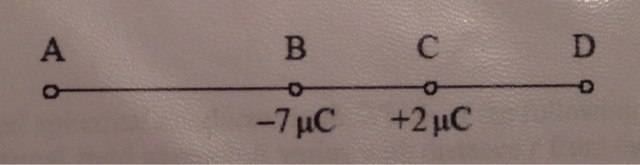# Zero electric field strength

Two isolated point charges -7μC and +2μC are at a fixed distance apart. At which point is is it possible for the electric field strength to be zero?I don't know where to start with only 2 numbers and no distances. I know that E= kQ/r^2

Simon Bridge
Homework Helper
Well ... if the two charges were equal, same charge, which point has zero field?
"Half way between them." right? You don't need distances to answer the question.

It kinda looks like they want you to pick point A or point D. That makes it easier.
One point is to the left and the there to the right - only one could plausibly have a zero field.
You get to do it either by understanding the physics or by doing the algebra with the distance being D.

Thanks for your help but I still don't understand. The answer is D but I can't see how you would know that with no numbers apart from the charges. I've tried calculating E using sample distances and get no where near zero. I want to understand the physics and the algebra but neither are making sense as yet.

. The answer is D but I can't see how you would know that with no numbers apart from the charges. I've tried calculating E using sample distances and get no where near zero. I want to understand the physics and the algebra but neither are making sense as yet.

Here's the idea:

Think about the negative charges as ''pullers'' and positive as ''pushers'' (owing to their electric field lines). Now, since -7μC has a greater magnitude, its pulling strength (or electric field strength) is higher than the pushing strength of 2μC, right?

So, to check the zero field intensity, position a charge in the points A and D and try to find out the position such that the pushing strength of 2μC just overcomes/balances the pulling strength of -7μC keeping in mind that the farther you go, lesser your field strength becomes.

Thank you, that's a really helpful way to picture it. I can see how it would be solved as a multiple choice question without calculations now. Thanks.

Chestermiller
Mentor
Two isolated point charges -7μC and +2μC are at a fixed distance apart. At which point is is it possible for the electric field strength to be zero?

View attachment 66245

I don't know where to start with only 2 numbers and no distances. I know that E= kQ/r^2
You can establish precisely where the field strength will be zero by expressing it in terms of the distance d between the charges (i.e., scaling to the distance between the charges).